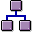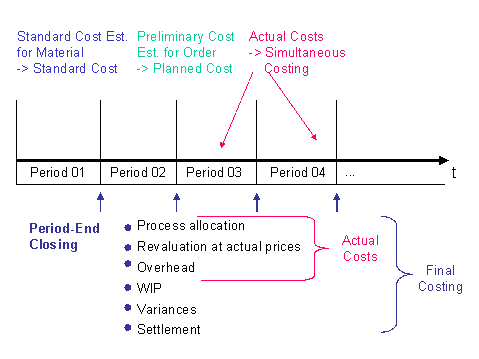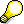Sequence of Steps in Cost Object Controlling: ScenarioIn the application component Product Cost Planning, you first create a standard cost estimate for the material to establish the standard cost.

Then the following sequence of steps is performed in the application component Cost Object Controlling:

1. Preliminary costing
2. The preliminary cost estimate calculates the planned cost for the object being costed. For example, if the cost object is a production order, planned costs can be used in variance calculation as the basis for calculating target costs.

3. Simultaneous costing
4. All actual costs incurred for a cost object are collected directly on the cost object. Actual costs are incurred in different ways, such as through internal activity allocations and material withdrawals. This process of collecting actual costs on a cost object is called simultaneous costing. Simultaneous costing enables you to view and analyze the actual costs on a cost object at any time.

5. Final costing

Once the cost object has been produced, final costing determines the actual costs incurred during the production process. Final costing also serves the purposes of cost analysis and control.

Final costing is normally performed as a period-end closing process. For this reason, final costing in the R/3 System is one of the menu options under Period-End Closing.

The period-end closing process includes the capture of period costs. A period cost is an actual cost that is not attributable to the cost object from a particular activity. This can be the revaluation of activities at actual prices or the calculation of process costs or overhead, for example.

In addition, the period-end closing process can determine the value of the unfinished goods (work in process).

You can calculate variances in the period-end closing process in Product Cost by Period and Product Cost by Order.

When you settle, you can transfer data to other application components such as Financial Accounting.

Period-end closing in Cost Object Controlling is performed after period-end closing in Cost Center Accounting.

Sequence of Steps in Cost Object ControllingThe above illustration shows how objects are handled in Cost Object Controlling.

In a make-to-stock environment, you normally create standard cost estimates for your materials in the Product Cost Planning component. These cost estimates calculate the standard costs of the materials. The sum of the standard costs can be written to the material’s master record as the standard price and used to valuate the material (see also: Basic Decisions in Cost Object Controlling).Note the special processing requirements for calculating standard costs and standard prices in sales-order-related production with a valuated sales order stock.

Planned costs for cost objects can be calculated in preliminary cost estimates. Planned costs can be calculated in:

At the end of each period, the cost objects are processed in the period-end closing function:

• The cost objects are debited with additional actual costs (period costs).
• The value of the unfinished products (work in process) or the variances can be calculated.
• Data can be sent to other application components.Note the exact meaning of the following terms:

• Standard costs
• Planned costs
• Target costs